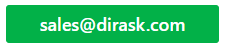Home
IT Knowledge
IT Career
Inspiration
Languages
EN

# JavaScript - async chain of operations with result

6 points
Created by:Greg
5140
 Have you ever needed to notify some logic about completed task and later go to next one with async code?

In this short article, we're going to have a look at how in JavaScript create async chain of operations that do not let execute next one before current one is completed, returning each time result of operation for chain node caller.

## `async/await` based example

Main advantages of this approach is: scheduling logic keeps current context of promise.

Note: inside `f1`, `f2` and `f3` functions we can use sync and async logic calling `await` to wait the job finish.

``````// ONLINE-RUNNER:browser;

let createChain = () => {
let chain = Promise.resolve();
return (action) => {
return new Promise(resolve => {
chain = chain.then(async () => resolve(await action()));
});
};
};

// Helper logic:

let sleep = (ms) => {
return new Promise(resolve => setTimeout(resolve, ms));
};

// Usage example:

let schedule = createChain();

let f1 = async () => {
console.log('1 sheduled');
await sleep(100);
console.log('1 finishing');
return 1;
};
let f2 = async () => {
console.log('2 sheduled');
await sleep(500);
console.log('2 finishing');
return 2;
};
let f3 = async () => {
console.log('3 sheduled');
await sleep(1000);
console.log('3 finishing');
return 3;
};

let doJobs = async () => {
let p3 = schedule(f3);
let p2 = schedule(f2);
let p1 = schedule(f1);

p1.then(r1 => console.log('> 1 done: result=' + r1));
p2.then(r2 => console.log('> 2 done: result=' + r2));
p3.then(r3 => console.log('> 3 done: result=' + r3));
};
doJobs();``````

Where: `f1` - function 1, `r1` - result 1, etc.

## Promise based example

Main disadvantage of presented approach is this section is need to assign byself  variables in proper way after each operation - keeping current context of promise.

Note: inside `f1`, `f2` and `f3` functions we can use sync and async logic calling `await` to wait the job finish.

``````// ONLINE-RUNNER:browser;

// Helper logic:

let sleep = (ms) => {
return new Promise(resolve => setTimeout(resolve, ms));
};

// Usage example:

let f1 = async () => {
console.log('1 sheduled');
await sleep(100);
console.log('1 finishing');
return 1;
};
let f2 = async () => {
console.log('2 sheduled');
await sleep(500);
console.log('2 finishing');
return 2;
};
let f3 = async () => {
console.log('3 sheduled');
await sleep(1000);
console.log('3 finishing');
return 3;
};

let chain = Promise.resolve();

let p3 = chain.then(f3);
p3.then(r3 => console.log('> 3 done: result=' + r3));

let p2 = p3.then(f2);
p2.then(r2 => console.log('> 2 done: result=' + r2));

let p1 = p2.then(f1);
p1.then(r1 => console.log('> 1 done: result=' + r1));``````

Where: `p1` - promise 1, `f1` - function 1, `r1` - result 1, etc.